Function Repository Resource:

# SubsetGroup

Compute a group induced by a permutation group on k-subsets

Contributed by: Wolfram Staff (original content by Sriram V. Pemmaraju and Steven S. Skiena)
 ResourceFunction["SubsetGroup"][g,s] returns the group induced by a group g of n-permutations acting on the set s of k-subsets of {1,…n}. ResourceFunction["SubsetGroup"][g,s,type] treats s as a set of k-subsets or k-tuples, depending on type.

## Details and Options

The group g can be specified as an abstract group or a permutation List {perm1,perm2,}.
ResourceFunction["SubsetGroup"] returns a PermutationGroup object.
type can be either "Ordered" or "Unordered".
The default value of type is "Unordered".
ResourceFunction["SubsetGroup"][g,s,"Ordered"] treats s as a set of k-tuples.

## Examples

### Basic Examples (2)

The permutation group induced on the set of all 2-subsets of {1,2,3} by the cyclic group C3:

 In:=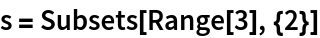Out=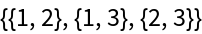In:=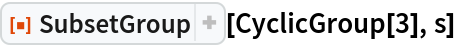Out=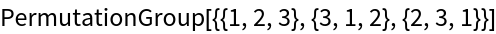The permutation group induced on the set of all 2-subsets of {1,2,3,4} by the cyclic group C4:

 In:=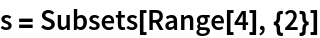Out=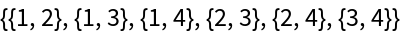In:=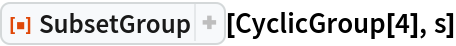Out=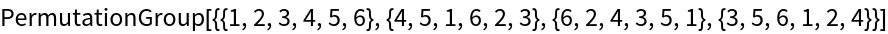### Scope (3)

Specify the group as a permutation List:

 In:=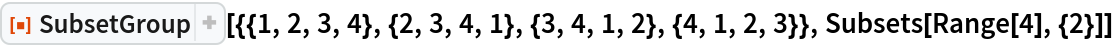Out=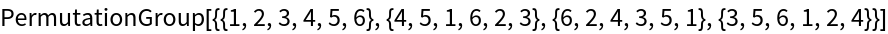Or an abstract group:

 In:=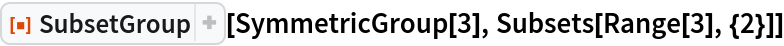Out=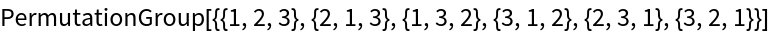The permutation group induced on the set of ordered 2-subsets by C4:

 In:=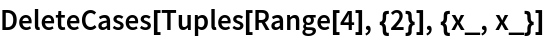Out=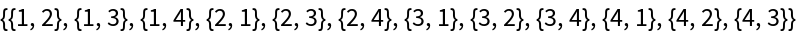In:=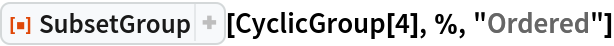Out=### Applications (3)

Permutation group on the edges of the 5-vertex wheel graph:

 In:=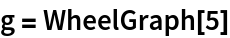Out=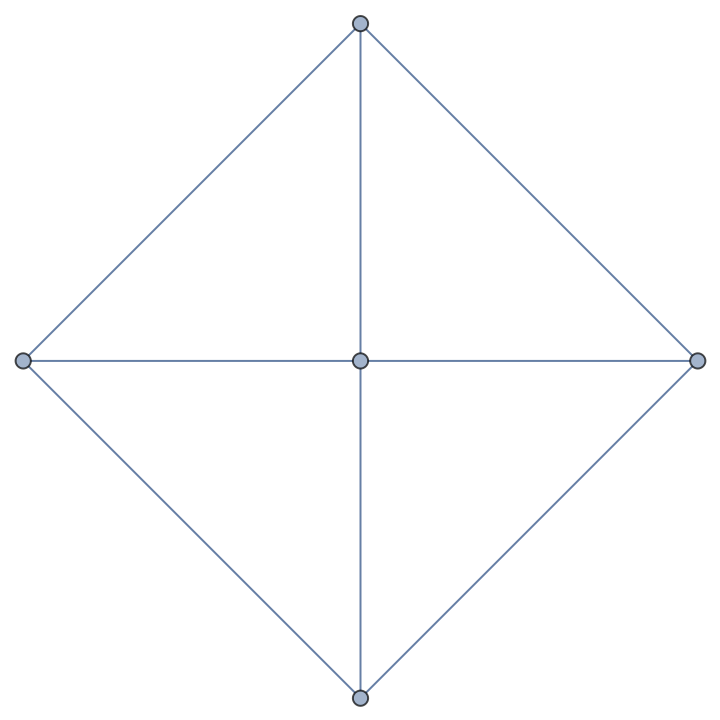In:=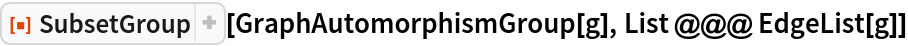Out=The number of colorings of 4-node simple graphs using at most n colors (OEIS A063842):

 In:=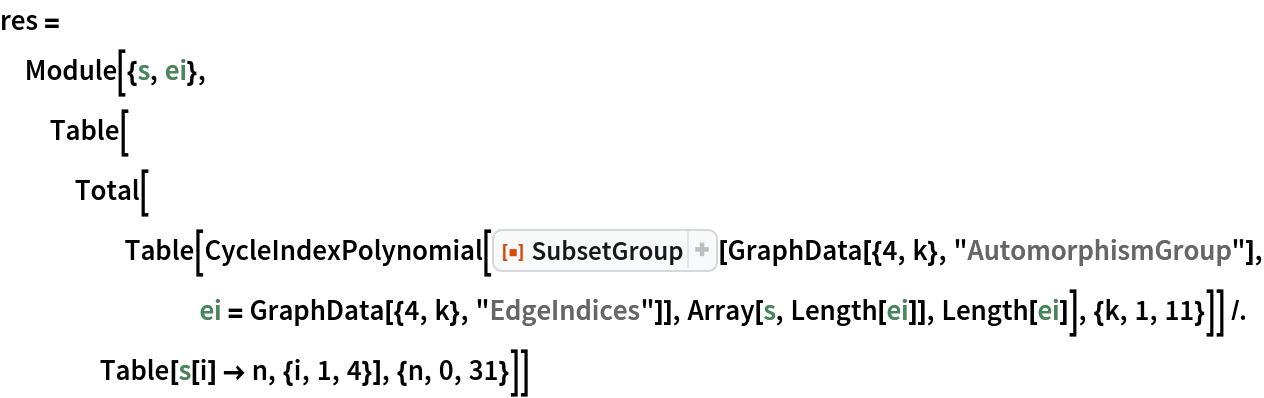Out=Count the number of distinct dice as orbit representatives of a permutation group induced by a group of symmetries acting on a set of faces of a cube:

 In:=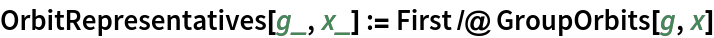In:=In:=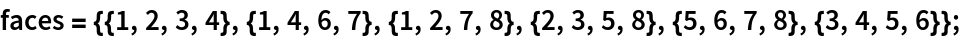In:=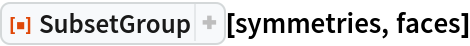Out=In:=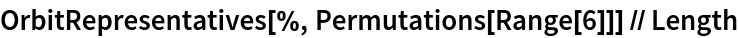Out=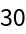### Properties and Relations (2)

n 2-subsets of {1,2,3,4,5,6} yield a permutation group of n-permutations:

 In:=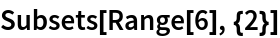Out=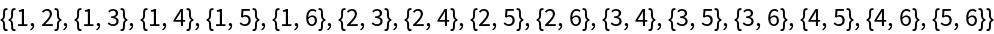In:=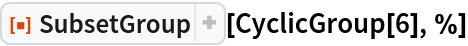Out=In:=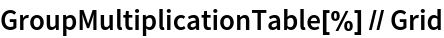Out=The n ordered 2-subsets of {1,2,3,4,5,6} yield a permutation group of n-permutations:

 In:=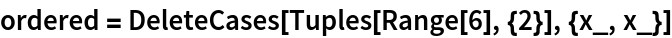Out=In:=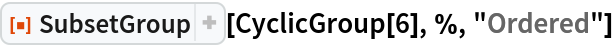Out=In:=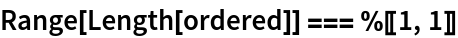Out=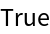## Version History

• 1.0.0 – 05 October 2020